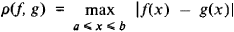# complete metric space

Also found in: Wikipedia.

## complete metric space

[kəm′plēt ¦me·trik ′spās]
(mathematics)
A metric space in which every Cauchy sequence converges to a point of the space. Also known as complete space.

## Complete Metric Space

a metric space in which the Cauchy convergence criterion is satisfied.

A sequence of points x1, x2, …, xn, … on a line, in a plane, or in space is said to be a Cauchy, or fundamental, sequence if for sufficiently large numbers n and m the distance between the points xn and xm becomes arbitrarily small. According to the Cauchy criterion, such a sequence of points has a limit if, and only if, it is a Cauchy sequence. For many sets of mathematical objects, such as functions or operators, a concept of distance can be introduced that has properties analogous to the properties of ordinary distance. The set is then said to be a metric space. The concept of the limit of a sequence of points in the metric space can be defined in the usual way. If the Cauchy criterion holds, the space is called complete.

Examples of complete metric spaces are Euclidean spaces and many other linear spaces—for example, the space of continuous functions on the interval [a, b] with distanceand Hubert space. A closed subset of a complete metric space is itself a complete metric space. If a metric space is not complete, it can be made complete in a way analogous to the way the set of rational numbers can be augmented by the irrationals to form the set of real numbers.

The concept of completeness can be extended to nonmetric topological spaces in which neighborhoods of different points can be compared. Examples of such spaces are topological groups and rings.

## complete metric space

(theory)
A metric space in which every sequence that converges in itself has a limit. For example, the space of real numbers is complete by Dedekind's axiom, whereas the space of rational numbers is not - e.g. the sequence a=1; a[n_+1]:=a[n]/2+1/a[n].
References in periodicals archive ?
The topics include Kyiv from the fall of 1943 through 1946: the rebirth of mathematics, two consequences of extension of local maps of Banach spaces: applications and examples, Hasse-Schmidt derivations and the Cayley-Hamilton theorem for exterior algebras, some binomial formulae for non-commuting operators, and the complete metric space of Riemann integrable functions and differential calculus in it.
If (X, d) is a complete metric space, then any element x in X is changed to x'(x' [member of] R) at function d: XxX[right arrow]R.
Let (X, d) be a complete metric space and T: X [right arrow] CB(X).
Let X be a complete metric space. Let [p.sub.1], ..., [p.sub.N] [member of] (0,1) such that [[summation].sup.N.sub.i=1] [p.sub.i] = 1.
Let X be a complete metric space and let [PHI] : X [right arrow] [(IFS).sup.X], [psi] : X [right arrow] X be ([alpha], [beta]) compatible mappings.
Among those generalizations, there is Kirk-Saliga fixed point theorem (see ) which states that any map T : X [right arrow] X has a fixed point provided that X is complete metric space and there exist an integer p [member of] N and a lower semicontinuous function [phi] : X [right arrow] [0, [infinity]) such that
introduced in  the notion of 0 - [sigma] complete metric space. This concept was further extended by Alghamdi et al.
Let (X,d) be a complete metric space and let T be a self-mapping on X satisfying (1).
Let (A, B) be a nonempty subset of a complete metric space (X, d).
Let (X,d) be a complete metric space, and let F : X [right arrow] X be a self-map.
A global NPC space is a complete metric space E = (E, d) for which the following inequality holds true: for each pair of points [x.sub.0], [x.sub.1] [member of] E there exists a point y [member of] E such that, for all points z [member of] E,
It states that if (X, d) is a complete metric space and f: X [right arrow] X satisfies: there exists [lambda] [member of] [0,1) such that

Site: Follow: Share:
Open / Close